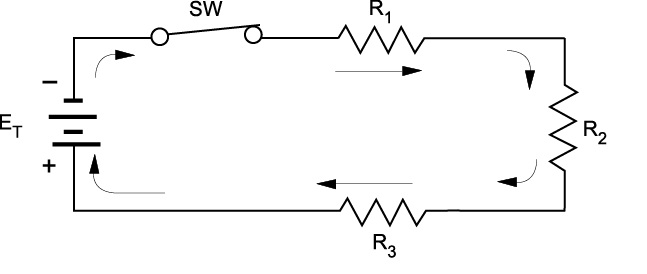# 7.1: Series Circuits

•• Camosun College
$$\newcommand{\vecs}{\overset { \rightharpoonup} {\mathbf{#1}} }$$ $$\newcommand{\vecd}{\overset{-\!-\!\rightharpoonup}{\vphantom{a}\smash {#1}}}$$$$\newcommand{\id}{\mathrm{id}}$$ $$\newcommand{\Span}{\mathrm{span}}$$ $$\newcommand{\kernel}{\mathrm{null}\,}$$ $$\newcommand{\range}{\mathrm{range}\,}$$ $$\newcommand{\RealPart}{\mathrm{Re}}$$ $$\newcommand{\ImaginaryPart}{\mathrm{Im}}$$ $$\newcommand{\Argument}{\mathrm{Arg}}$$ $$\newcommand{\norm}{\| #1 \|}$$ $$\newcommand{\inner}{\langle #1, #2 \rangle}$$ $$\newcommand{\Span}{\mathrm{span}}$$ $$\newcommand{\id}{\mathrm{id}}$$ $$\newcommand{\Span}{\mathrm{span}}$$ $$\newcommand{\kernel}{\mathrm{null}\,}$$ $$\newcommand{\range}{\mathrm{range}\,}$$ $$\newcommand{\RealPart}{\mathrm{Re}}$$ $$\newcommand{\ImaginaryPart}{\mathrm{Im}}$$ $$\newcommand{\Argument}{\mathrm{Arg}}$$ $$\newcommand{\norm}{\| #1 \|}$$ $$\newcommand{\inner}{\langle #1, #2 \rangle}$$ $$\newcommand{\Span}{\mathrm{span}}$$$$\newcommand{\AA}{\unicode[.8,0]{x212B}}$$

A series circuit is constructed by connecting all of the circuit components in line with one another. The schematic diagram in Figure $$\PageIndex{1}$$ is an example of a simple series circuit. In this case, a battery (source) is connected through a switch to three resistors (load devices), all of which are in line with one another.Figure $$\PageIndex{1}$$: A simple series circuit (CC BY-NC-SA; BC Industry Training Authority)

When the switch is closed, there is only one path for current flow. Any circuit that provides only one path for current flow is categorized as a series circuit.

If any part of a series circuit is opened, the current cannot flow and none of the components will operate. The circuit may be opened by the switch or by the failure of a component in the circuit. For example, some decorative lights have clusters within the string that are connected as a series circuit. If one lamp burns out (or opens), all the other lamps go out. You then have to test each lamp individually to find the failed bulb, and this gets very challenging if two bulbs happen to be damaged.

## Application of series circuits

Electrical components or devices are generally connected in series whenever it is necessary to:

• control the amount of current flow in a circuit
• divide the total voltage of a supply

For example:

• Switches are connected in series with loads so that you can energize or de-energize different loads.
• Protective devices such as fuses and overload relays are connected in series with line conductors.
• By connecting equal values of resistance in series, the same voltage drop can be obtained across each resistance. Twenty Christmas tree lights connected in series to a 120 V supply would have a voltage rating of 6 V per light.